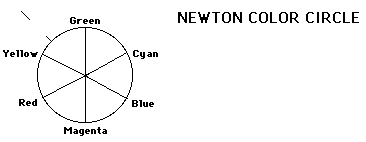# Light Box Experiments

These are a few exercises to get you to explore the capabilities of the Light Box. The boxes are to be powered from the DC supply terminals on your lab table.

The instructions are general, so that your team can explore to find a way to accomplish the task with the equipment included.

1. Make a rough determination of the focal lengths of the two converging and one diverging lenses with the symmetric curved edges.

2. Devise a lens combination that takes a wide parallel beam of light and converts it into a narrow beam of light, also parallel. Describe the combination you develop, identifying the lenses used and making whatever measurements are needed.

3. Examine rays of light through a rectangular strip of clear material . Make a sketch of the directons of the rays.

4. Work your way around the Newton color circle, using the color slides which are a part of the kit. Examine the colors produced when you use colored slides which are two positions apart. In equal mixture, these should give the color between them. Comment on the color you perceive - is it what it is supposed to be?5. Make a rough determination of the focal length of one of the curved mirrors. Then measure the radius of curvature of the mirror. How do they compare (calculate their ratio)?

Index
HyperPhysics***** Physics 7120 ***** Physics 7120 Laboratory Go Back